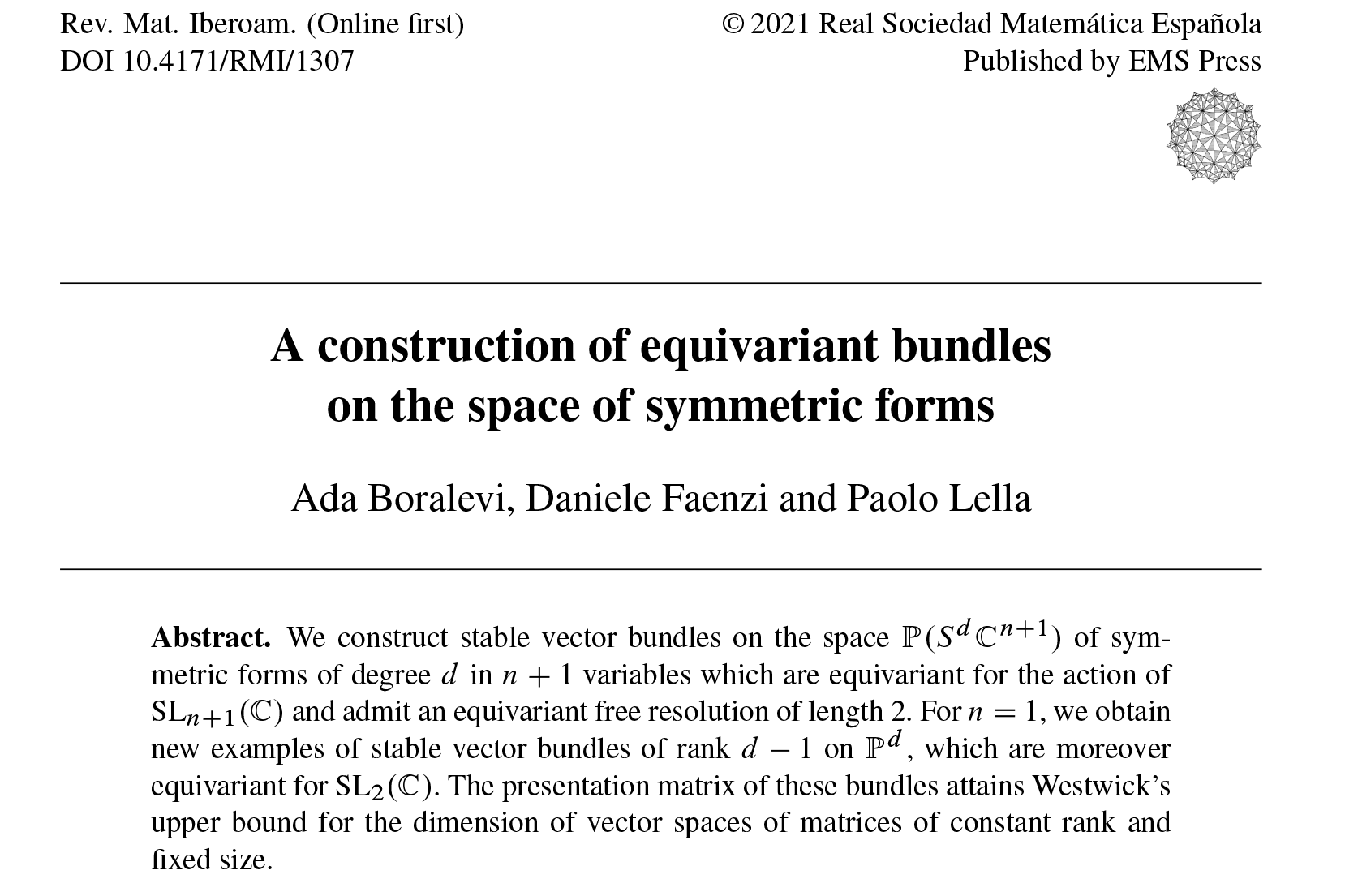### A construction of equivariant bundles on the space of symmetric forms

##### Accepted on Revista Matemática Iberoamericana, 2021.
DOI: 10.4171/RMI/1307

Keywords

Abstract. We construct stable vector bundles on the space of symmetric forms of degree $d$ in $n+1$ variables which are equivariant for the action of $\text{SL}_{n+1}(\mathbb{C})$, and admit an equivariant free resolution of length 2. For $n=1$, we obtain new examples of stable vector bundles of rank $d-1$ on $\mathbb{P}^d$, which are moreover equivariant for $\text{SL}_2(\mathbb{C})$. The presentation matrix of these bundles attains Westwick’s upper bound for the dimension of vector spaces of matrices of constant rank and fixed size.

© 2021 GAA@polimi Generated with Hugo and inspired by Resume theme Last update: June 8, 2022